Published

# MEGR 3171 Engine Sound, Acceleration, and Temperature Sensor

This sound system will measure the temperature, acceleration, and sound emitted from any engine.

IntermediateFull instructions provided4 hours20## Things used in this project

### Hardware componentsJumper wires (generic)
×11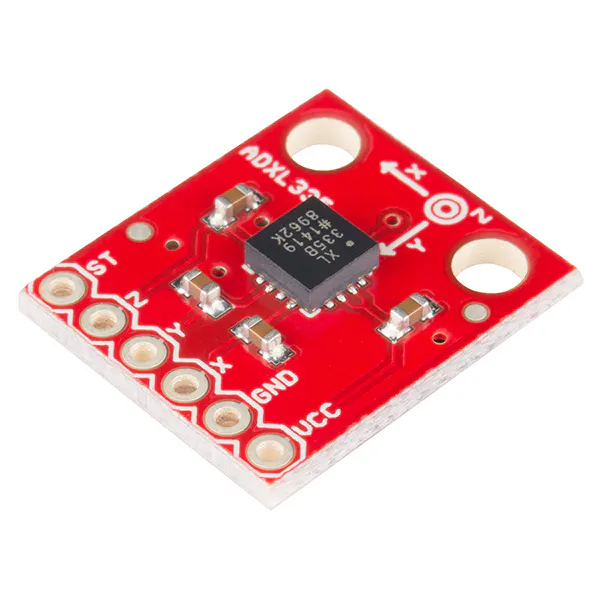SparkFun Triple Axis Accelerometer Breakout - ADXL335
×1
 DFRobot DFR0034 Sound Sensor
×1
 Amazon Web Services NTC 10KOhm Thermistor
×1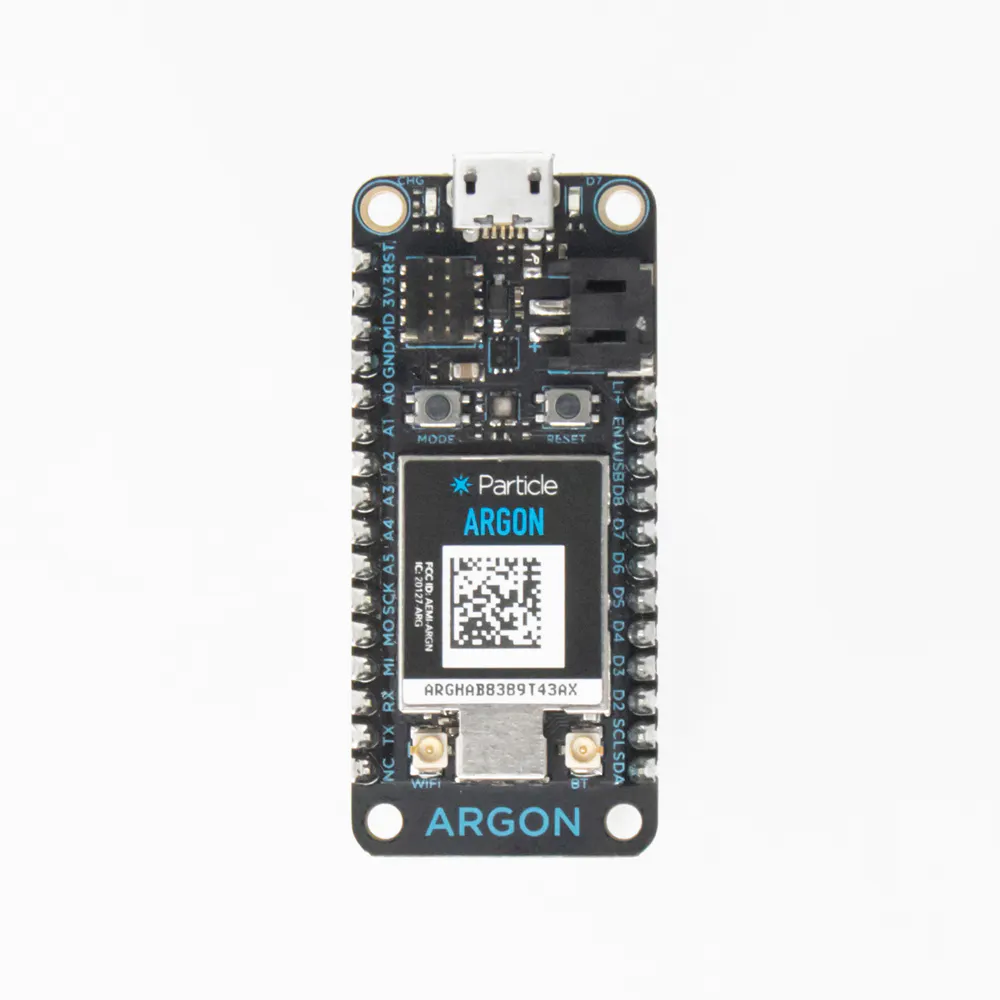Particle Argon
×3×3

### Software apps and online servicesParticle Build Web IDEThingSpeak API
 Fritzing

## Schematics

### Accelerometer Fritzing Circuit Diagram

This is the diagram of how the accelerometer was connected to the Particle Argon. The accelerometer ADXL335 had six connections. The VCC was connected to the 3V3 pin on the Argon. The GRD was connected to the Argon GRD pin. The X pin was connected to the A1 Argon pin. The Y and Z pins were connected to the A2 and A3 pins on the Argon respectively.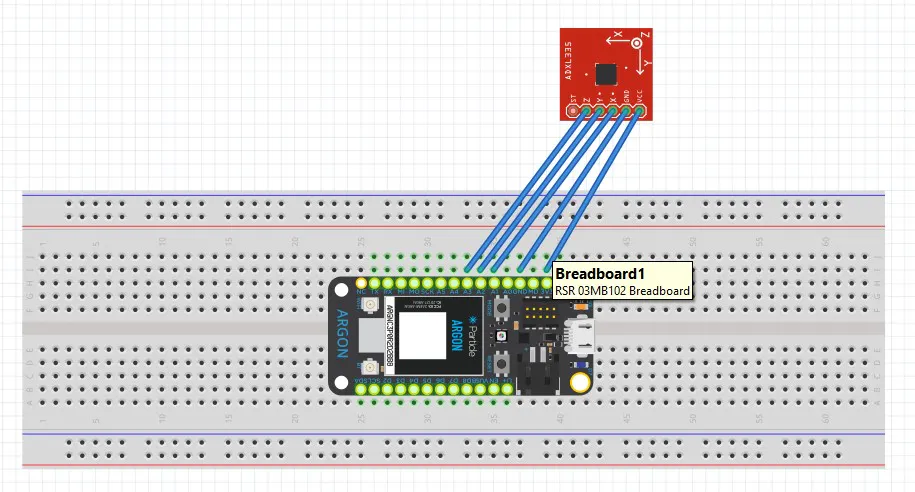### Thermistor Fritzing Circuit Diagram

This is the circuit diagram for the temperature sensor connected to the second Argon. In this circuit, a 10 KOhm Resistor was used, as well as the 20KOhm NTC Thermistor. As seen in the diagram, the resistor was connected on one leg to both the thermistor and the A3 pin of the Argon. The second resistor leg was connected to the ground pin of the Argon. The other leg of the thermistor was connected to the 3V3 Argon pin in order to give power to the circuit.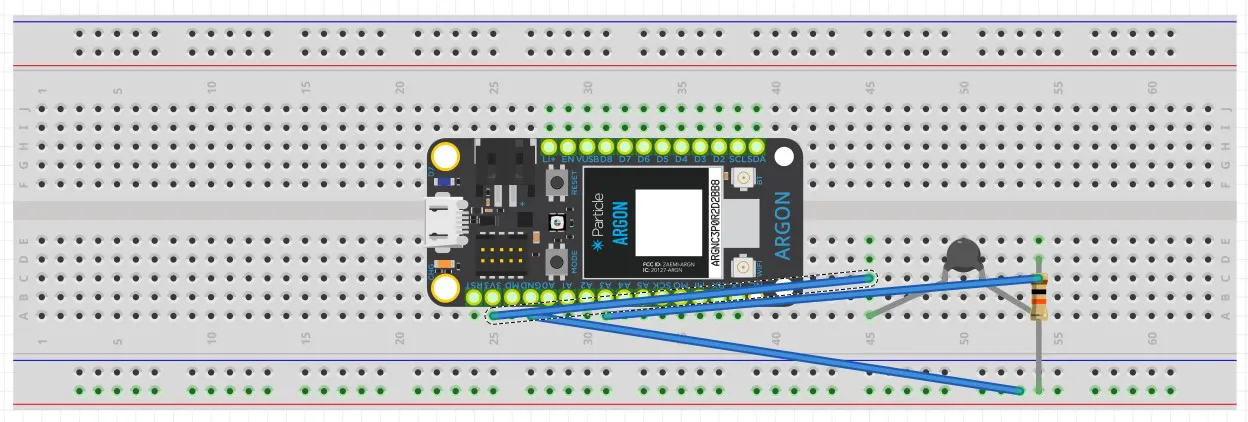### Sound Sensor Fritzing Circuit Diagram

This sound sensor DFR0034 was used in order to intake any sound data that may come from the car's exhaust or engine. There were only three pins that were on the sound sensor that needed connection to the third Particle Argon. The VCC pin is connected to the 3V3 pin on the Argon in order to give power to the circuit. The GND pin then was connected to the GND pin on the Argon. The final sensor pin with the output was connected to the A0 Argon pin.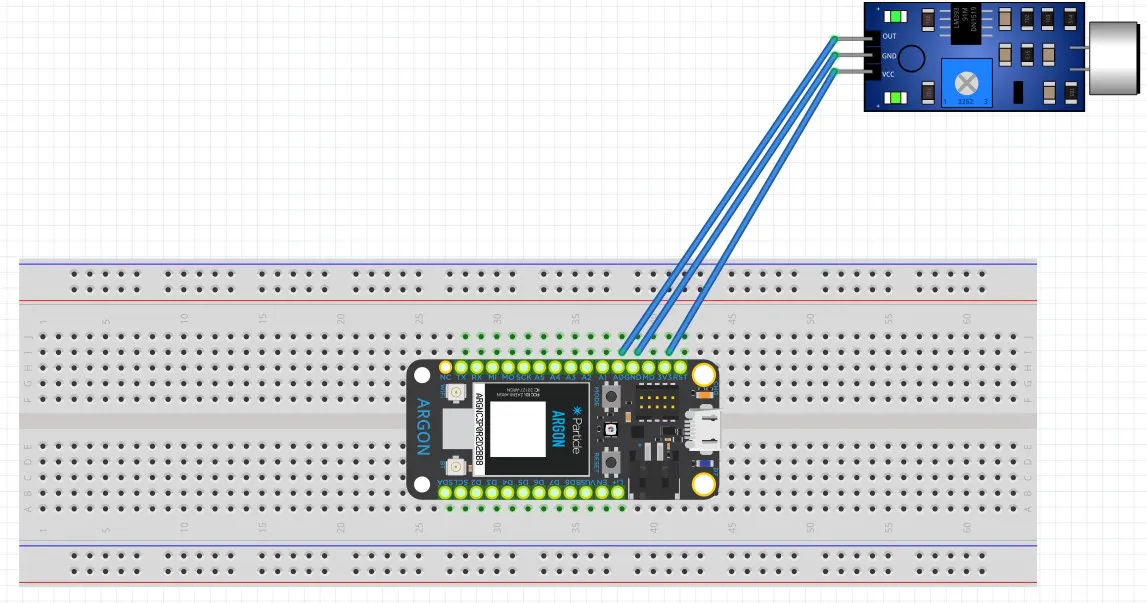## Code

### Accelerometer Sensor Code

C/C++
This code has a twofold purpose. It first is coded to read the sensor data and output specific values if the sensor is connected correctly. The second purpose of this code is to take all the data that is recorded from the acceleration sensor and transfer the data onto graphs on ThingSpeak.
```// This #include statement was automatically added by the Particle IDE.
#include <ThingSpeak.h>

TCPClient client;

unsigned long myChannelNumber = 1571995;
const char * myWriteAPIKey = "4OF8C29D3MSP8SOY";

const int x_out = A1; /* connect x_out of module to A1 of Particle Photon board */
const int y_out = A2; /* connect y_out of module to A2 of Particle Photon board */
const int z_out = A3; /* connect z_out of module to A3 of Particle Photon board */

void setup()
{
// Subscribe to the integration response event
Particle.subscribe("hook-response/Project3171_", myHandler, MY_DEVICES);
}

void myHandler(const char *event, const char *data) {
// Handle the integration response

Serial.begin(9600);

}
void loop() {

double x_g_value, y_g_value, z_g_value;
double roll, pitch, yaw;

x_adc_value = analogRead(x_out); /* Digital value of voltage on x_out pin */
y_adc_value = analogRead(y_out); /* Digital value of voltage on y_out pin */
z_adc_value = analogRead(z_out); /* Digital value of voltage on z_out pin */

Serial.print("x = ");
Serial.print("\t\t");
Serial.print("y = ");
Serial.print("\t\t");
Serial.print("z = ");
Serial.print("\t\t");

x_g_value = ((((double)(x_adc_value * 3.3) / 4095) - 1.65) / 0.330);
y_g_value = ((((double)(y_adc_value * 3.3) / 4095) - 1.65) / 0.330);
z_g_value = ((((double)(z_adc_value * 3.3) / 4095) - 1.65) / 0.330);

roll = (((atan2(y_g_value , z_g_value) * 180) / 3.14) + 180);
pitch = (((atan2(z_g_value , x_g_value) * 180) / 3.14) + 180);

String data = String(roll);

const char * eventName = "Project3171_";
unsigned long myChannelNumber =1571995;
const char * myWriteAPIKey = "4OF8C29D3MSP8SOY";
// Particle.publish(eventName, "{ \"Roll\": \"" + String(roll) + "\", \"Pitch\": \"" + String(pitch) + "\", \"key\": \"" + myWriteAPIKey + "\" }", PRIVATE);
Particle.publish(eventName, "{ \"Roll\": \"" + String(roll) + "\", \"Pitch\": \"" + String(pitch) + "\"}", PRIVATE);

}
```

### Temperature Sensor Code

C/C++
This code also has a twofold purpose. It first is coded to read the sensor data and output specific values if the sensor is connected correctly. The second purpose of this code is to take all the data that is recorded from the temperature sensor and transfer the data onto graphs on ThingSpeak.
```// This #include statement was automatically added by the Particle IDE.
#include <ThingSpeak.h>

TCPClient client;

unsigned long myChannelNumber = 1571995;
const char * myWriteAPIKey = "4OF8C29D3MSP8SOY";

const int thermistor_output = A3;
void setup() {

// Subscribe to the integration response event
Particle.subscribe("hook-response/Project3171_", myHandler, MY_DEVICES);
}

void myHandler(const char *event, const char *data) {
// Handle the integration response

Serial.begin(9600);

double temperature;
}

void loop() {
double output_voltage, thermistor_resistance, therm_res_ln, Temperature;

output_voltage = ((thermistor_adc_val * 3.3)/ 4095.0);

thermistor_resistance = ((3.3 * (10/output_voltage))-10);

thermistor_resistance = thermistor_resistance * 1000;

therm_res_ln = log(thermistor_resistance);

Temperature = (1/(0.001129148 + (0.000234125 * therm_res_ln)+( 0.0000000876741 * therm_res_ln * therm_res_ln)));

Temperature = Temperature - 273.15;
Temperature = ((Temperature*1.8) + 32)-15;

String data = String(Temperature);
delay(10000);

Serial.print("Temperature=");
Serial.print(Temperature);
Serial.print("\n\n");

const char * eventName = "Project3171_";
unsigned long myChannelNumber =1571995;
const char * myWriteAPIKey = "4OF8C29D3MSP8SOY";
Particle.publish(eventName, "{ \"Temperature\": \"" + String(Temperature) + "\", \"key\": \"" + myWriteAPIKey + "\" }", PRIVATE, NO_ACK);

delay(10000);
}
```

### Sound Sensor Code

C/C++
This code also has a twofold purpose. It first is coded to read the sensor data and output specific values if the sensor is connected correctly. The second purpose of this code is to take all the data that is recorded from the sound sensor and transfer the data onto graphs on ThingSpeak.
```// This #include statement was automatically added by the Particle IDE.
#include <ThingSpeak.h>

int sensorPin = A0; //analog --> most helpful when recording realtime data!!
int Decibel = 0; //define variable to store analog value

TCPClient client;

unsigned long myChannelNumber = 1571995;
const char * myWriteAPIKey = "4OF8C29D3MSP8SOY";

const int sound_output = A0;

void setup()  {

// Subscribe to the integration response event
Particle.subscribe("hook-response/Project3171_", myHandler, MY_DEVICES);
}

void myHandler(const char *event, const char *data) {
// Handle the integration response

Serial.begin(9600);
}
void loop()
{
Serial.println(String(Decibel) );//print analog value onto serial monitor
delay(5000);
String data = String(Decibel);

const char * eventName = "Project3171_";
unsigned long myChannelNumber =1571995;
const char * myWriteAPIKey = "4OF8C29D3MSP8SOY";
// Particle.publish(eventName, "{ \"Roll\": \"" + String(roll) + "\", \"Pitch\": \"" + String(pitch) + "\", \"key\": \"" + myWriteAPIKey + "\" }", PRIVATE);
Particle.publish(eventName, "{ \"Decibel\": \"" + String(Decibel) + "\", \"key\": \"" + myWriteAPIKey + "\"}", PRIVATE, NO_ACK);

delay(5000);
}
```

## Credits

### Natalie Elder

0 projects • 0 followers

### Eddie Scott

0 projects • 1 follower

### Jon Tresidder

0 projects • 1 follower